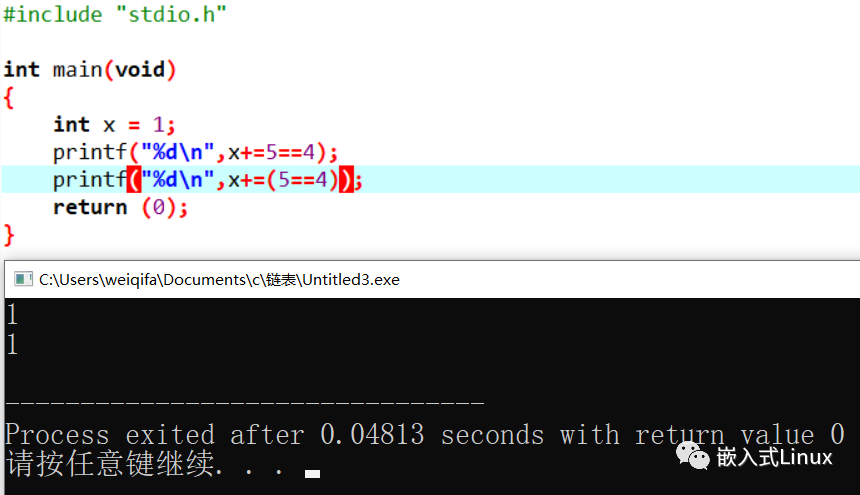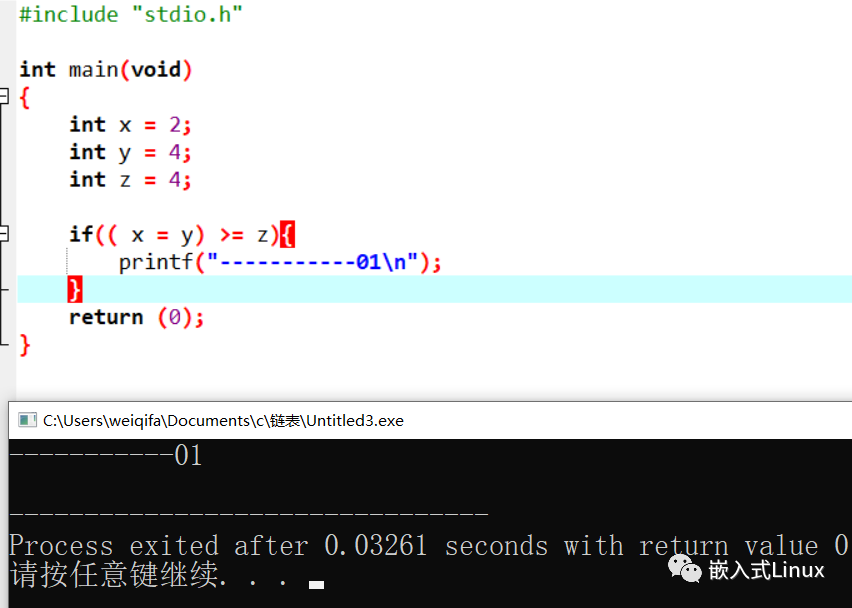## 【转】C 语言中，x += 5 == 4 是什么意思？2020-05-19 10:17:55    评分

#讨论这个有意义吗？

`((x += 5) == 4)`

`(x += （5 == 4)）`

C语言的标准有几百页，大部分人不会仔细研究每个细节，有些工程师觉得某些东西没有用，大概率是因为他在自己的工作中没有用到。有的程序员喜欢研究或者在工作中需要使用这样的方法，这就是这种代码存在的其中一个原因吧。

#表达式和运算符优先级

C语言在一个表达式中，如果遇到几个运算符，要考虑优先级和结合方向。

• (x pri1 y )pri2 z

• x pri1 (y pri2 z)

a = b = c  表达式的意义是 a =（ b = c  ）

a + b + c  表达式的意义是 （a + b） + c

#C语言运算符优先级

#解析上面的表达式

`x += 5 == 4`

「+=」 的优先级是14

「 ==」 的优先级是 7

`x += (5 == 4)`#表达式的巧用```#include "stdio.h"
int main(void)
{
int x = 2;
int y = 4;
int z = 4;
if( x = y >= z){
printf("-----------01\n");
}
return (0);
}```